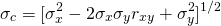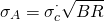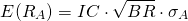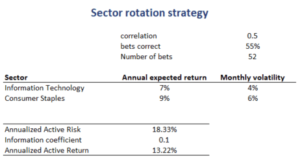# Sector Rotation Strategy

A sector rotation strategy is a market timing strategy that overweights certain sectors over time. For example, an active fund manager may overweight sectors he or she expects will outperform and underweight sectors he or she expects to underperform. While the approach is easy to implement, we need to know the information ratio and the breadth of the strategy. These will determine how much active risk we should allocate to the sector rotation strategy.

On this page, we consider a simple case where a manager engages in a pair trade. That is, the fund manager buys one sector and sells the other. We implement a numerical sector rotation strategy example in Excel. The spreadsheet is available for download at the bottom of the page.

## Sector rotation strategy formula

Let’s assume there are two sectors, sector X and sector Y. We have expected returns for both sectors equal to R(x) and R(y). We also have the volatility of both sectors as well as the correlation between the two sectors. The correlation between the two sectors is an important part that will determine the overall amount of risk of the sector rotation strategy.

We can calculate the active risk of the overall portfolio c as followsThe annualized active risk that should be taken is a function of the number of bets that are made during the year. The number of bets is measured using breadth (BR). If the manager rotates every month, then BR = 12.

We can calculate the appropriate annualized level of active risk asThe active return of this strategy will be## Sector rotation strategy example

Let’s consider an example to clarify the above formula. The figure below reports the results of a numerical example that implements all of the above formulas. While the formulas look challenging, it’s clear that this method is easy to implement.## Summary

We discussed a simple rotation strategy with two sectors. To be able to implement the approach, the fund manager needs to have an idea on the expected information ratio of the strategy and the frequency with which he or she will implement the strategy. These factors determine the amount of active risk that should be allocated to the strategy.

### Rotation strategy

Want to have an implementation in Excel? Download the Excel file: Sector Rotation